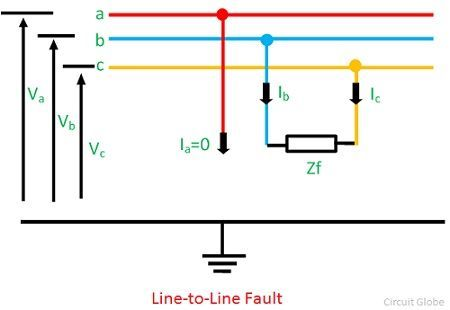# Line-to-Line Fault

A line to line fault or unsymmetrical fault occurs when two conductors are short circuited. In the figure shown below shows a three phase system with a line-to-line fault phases b and c. The fault impedance is assumed to be Zf. The LL fault is placed between lines b and c so that the fault be symmetrical with respect to the reference phase a which is un-faulted.The symmetrical components of a fault current in phase ‘a’ at the fault point can be divided into three component. The zero sequence component of current at phase a isIn the equation(1) Ib = -Ic. Positive sequence component of phase a is expressed asand the negative sequence component of phase a is given by the equation,The sequence current can also be found by matrix methodExpressing Va, Vb and Vc regarding voltages at the fault point are found by the relations given byCombination of equation (1), (4) and (5) givesThe sequence current of voltage at the fault point are determined by the relations shown belowCombination of equation (4), (10) and (9) givesThe fault current is given by the equationFrom equation (1) it is clear that the line-to-line fault the zero sequence component of current Ia0 is equal to zero. Equation (4) shows that the positive-sequence component of current is opposite in phase to the negative-sequence component of current.